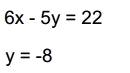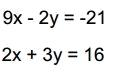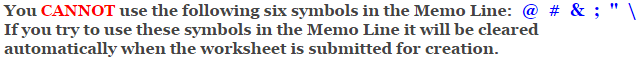# Algebra 2 - Systems of Equations Worksheets

## Solving Graphically Two Variable Systems of Equations Worksheets

This algebra 2 systems of equations worksheet will produce problems for solving two variable systems of equations graphically. You may select which type method the student should use to solve the problems. This algebra 2 systems of equations worksheet will produce eight problems per page.

### Type of Problems to Use

 Standard Form Slope-Intercept Form### Language for the Systems of Equations Worksheet

 English German Albanian Spanish Swedish Italian French Turkish Polish Norwegian

### Memo Line for the Systems of Equations Worksheet

You may enter a message or special instruction that will appear on the bottom left corner of the Systems of Equations Worksheet.### Systems of Equations Worksheet Answer Page

Now you are ready to create your Systems of Equations Worksheet by pressing the Create Button.

 Recommended Videos

If You Experience Display Problems with Your Math WorksheetMath-Aids.Com.  All rights reserved. Algebra 2 - Systems of Equations Worksheets